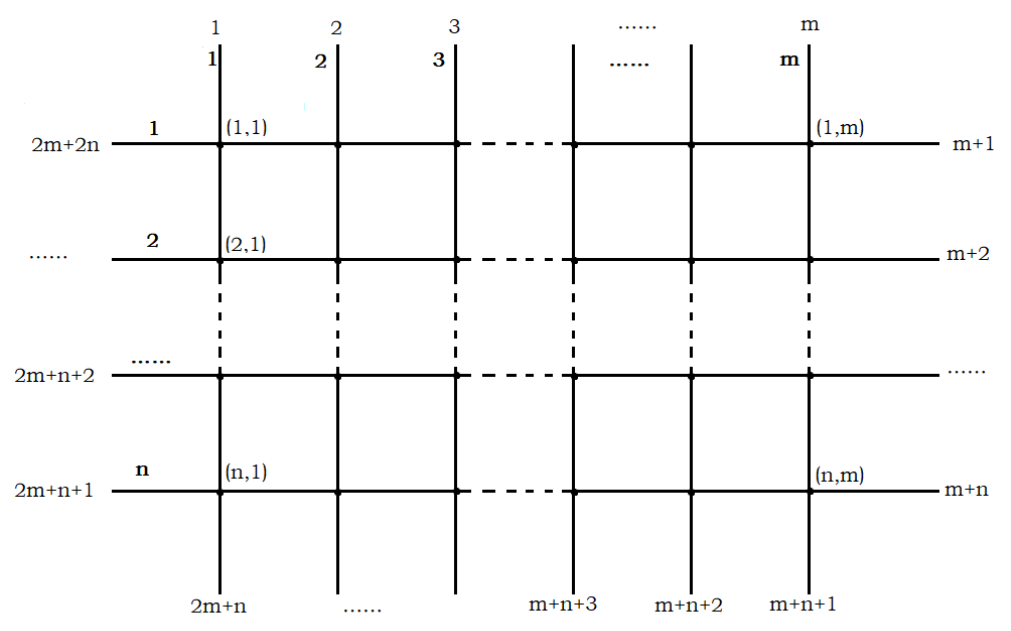# #687. 【CSP-S 2021】交通规划### 样例一

#### input

2 3 1
9 4 7
3 8
10 5
2
19 3 1
17 9 0



#### output

12



### 限制与约定

$1 \sim 2$ $5$ $50$
$3 \sim 5$ $18$ $2$
$6 \sim 8$ $18$ $50$
$9 \sim 10$ $100$ $2$
$11 \sim 12$ $100$ $50$
$13 \sim 16$ $500$ $2$
$17 \sim 20$ $500$ $50$